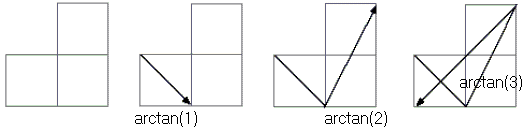# Trigonometric Identities with Arctangents

### John Molokach January 2, 2012

Five trigonometric identities can be easily observed in the following diagram:Here are the identities:

arctan(1) + arctan(2) + arctan(3) = π
arctan(1/2) + arctan(1/3) = π/4
arctan(1) + arctan(1/2) + arctan(1/3) = π/2
arctan(1) + arctan(1/2) = arctan(3)
arctan(1/3) + arctan(1) = arctan(2).

Do you see them all?